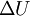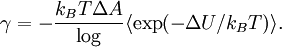# Test area method

(diff) ← Older revision | Latest revision (diff) | Newer revision → (diff)
Related to the test volume method for the pressure (which, in turn, is related to Widom test-particle method). The surface tension of a planar interface is given by the change in internal energy$\Delta U$ caused by "squeezing" the system: modifying both the length in the direction normal to the interface and the area in the plane of the interface, in such a way that the total volume is left unchanged.$\gamma = - \frac{ k_B T \Delta A } \log\langle \exp(-\Delta U/k_B T)\rangle.$